﻿ Why Galaxies Look Like Islands in the Universe- and on the Origin of Dark Matter and Energy

### Why Galaxies Look Like Islands in the Universe- and on the Origin of Dark Matter and Energy

Arne BergstromOPEN ACCESSPEER-REVIEWED

## Why Galaxies Look Like Islands in the Universe- and on the Origin of Dark Matter and Energy

Arne BergstromB&E Scientific Ltd, Seaford BN25 4PA, United Kingdom

### Abstract

The Boltzmann transport equation is the rigorous continuity equation for quantum propagation in space. Requiring only that this equation should be Lorentz-covariant and dispersion-free leads to an exponentially expanding universe, and also to an exponential increase with time of the number of quanta involved, which could explain the existence of the dominating proportion (95.1 %) of dark matter and dark energy observed in the universe. Simulations of the quantum propagation from the Pareto distribution and quantum duplication thus derived from Lorentz-covariance show two specific, distinct distributions: (1) dense clusters of quantum trajectories close to each other (“dark matter”?), and (2) an extensive web of extremely long trajectories (“dark energy”?) to new such clusters. The dark matter clusters thus form a large-scale distribution of galaxy-type islands separated from each other, and immersed in a dominating web of dark energy trajectories. The simulations performed also give a ratio of dark energy to dark matter in the universe, which is consistent with the ratio recently measured by the Planck Space Telescope.

### At a glance: Figures

12
Prev Next

• Bergstrom, Arne. "Why Galaxies Look Like Islands in the Universe- and on the Origin of Dark Matter and Energy." International Journal of Physics 3.2 (2015): 52-57.
• Bergstrom, A. (2015). Why Galaxies Look Like Islands in the Universe- and on the Origin of Dark Matter and Energy. International Journal of Physics, 3(2), 52-57.
• Bergstrom, Arne. "Why Galaxies Look Like Islands in the Universe- and on the Origin of Dark Matter and Energy." International Journal of Physics 3, no. 2 (2015): 52-57.

 Import into BibTeX Import into EndNote Import into RefMan Import into RefWorks

### 1. Introduction

A fundamental property of the world we live in is Lorentz covariance. Lorentz covariance can be interpreted as a prerequisite for us to be able to make consistent observations of the world around us. In particular, the requirement that the fundamental, exact continuity equation for quantum propagation in space - the Boltzmann transport equation - should be Lorentz-covariant can be shown [1, 2, 3, 4] to lead to the following basic characteristics of our world:

1. Lorentz-covariance of quantum propagation requires the universe to appear subjected to an exponentially accelerated expansion [2, 4].

2. This exponentially accelerated expansion is analogous  to a perspective in the time dimension of spacetime of the same type as ordinary perspective is in the space dimesions of spacetime.

3. Lorentz-covariance requires the universe to appear to contain a dominating background of some unknown dark energy/dark matter caused by a forced, apparent quantum doubling at each quantum collision with the surrounding medium [1, 2, 4]. This forced, exponential quantum creation may keep the average mass-energy density of the universe constant, balancing the apparent exponential expansion in (1).

4. Lorentz covariance requires the quanta involved in this process to appear [1, 2] to propagate with a mean free path described by a Pareto distribution, which thus for small path lengths is essentially an exponentially decreasing distribution, but which has a dominating tail that corresponds to a long-range, inverse quadratic distribution (see Figure 1 and Figure 2).

5. The blackbody spectrum of the cosmic microwave background radiation can be exactly described [3, 4] as an effect of this apparent, exponentially accelerated expansion of the universe.

It should be emphasised that these properties of the universe are strict consequences of the relativistic covariance of the Boltzmann continuity equation for the angular flux of particles, as will be described below. The requirement of Lorentz covariant quantum transport thus leads to an exponential expansion of the universe with an apparent quantum doubling at each collision with the surrounding medium, and to a Pareto distribution for the apparent path length, thus with an infinite mean distance to the next collision point due to the properties of the Pareto distribution.

A doubling of the particle number of this kind very quickly leads to enormous numbers. After only around 270 such doublings, 2270 ≈ 1080, we thus get the estimated [5, 6] total number of dark energy and dark matter quanta in the observable universe, which together correspond to some ninety-five percent of its total mass-energy .

A characteristic property of the Pareto distribution mentioned above is that for small path lengths it is essentially an exponentially decreasing function, but that its tail then corresponds to an inverse square law (see Figure 1 and Figure 2), thus with equal probability for every successive spherical shell. The Pareto distribution thus leads to a high probability of collisions very close to a source, but then also leads to a dominating number of collision points extremely far away. If the collision points close to the source correspond to new particles being created in a galaxy, then the distant collision points correspond to embryos to sister galaxies being created very far away, as illustrated in Figure 3 and Figure 4.

The quanta in the above scheme are thus required by relativistic covariance to have two characteristics: 1) the collision points (black) where the quantum doubling occurs, and 2) the occasionally very long paths (red) to new such clusters of collision points.

In the following, the close web of trajectories near collision points, and near to which new collision points may accumulate, will be interpreted as dark matter, while the extensive web of trajectories leading very far away and along which new clusters of collision points may potentially be created, will be interpreted as dark energy. Both forms obviously correspond to a mass-energy source, but the web of short trajectories (“dark matter”, 26.8 %  of the total mass of the universe) tends to cluster in smaller regions, whereas the web of far trajectories (“dark energy”, 68.3 %  of the total mass) tends to form a fabric encompassing the entire volume. The collision points themselves may represent “ordinary”, localised matter (4.9 %  of the total mass of the universe).

This is thus how the separate islands formed by stars as galaxies may relate to dark matter/dark energy in the universe, as will be further discussed in the following.

Figure 1. Pareto distribution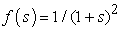compared to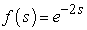, coinciding for small. Note the frequent occurrence of longer path lengths s in the Pareto distribution (cf Figure 2)
Figure 2. The Pareto distribution and negative exponential distribution in Figure 1 displayed in a loglog diagram in comparison with an inverse square distribution. The Pareto distribution agrees with a negative exponential for small and with an inverse square law for large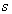### 2. The Pareto Distribution

The Pareto distribution of the quantum transport discussed above can be derived as follows.

The time-dependent propagation of neutral quanta (such as, e g, in gamma radiation) moving with the velocity of light c through a medium with which they interact by localized collisions, is rigorously described by the Boltzmann transport equation  given as follows,(1)

whereis a source term, and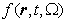is the angular flux in directionat point= (x, y, z) and time t. Possible interactions with the medium, through which the quanta propagate, are described by the interaction cross sectionand where the kernelthen describes how quanta may become scattered from directionto direction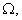and/or partially absorbed or multiplied (like neutrons in fission) in the process. It should be emphasized that the Boltzmann transport equation is a rigorous continuity equation for the angular flux, and is exact as long as the angular flux is sufficiently low so that the effects of particle-particle interactions between the propagating quanta themselves can be neglected.

The total fluxis defined from the angular flux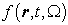as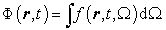(2)

For quanta undergoing isotropic scattering in a homogeneous medium, the quantum propagation as described by the fluxin (2) can be derived [1, 2] rigorously from the Boltzmann transport equation (1) to take the form of the “telegrapher’s equation” [9, 10],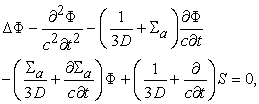(3)

where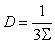(4)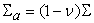(5)

with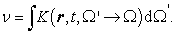(6)

Due to the third and fourth terms on the left-hand side of the telegrapher’s equation (3) above, this equation will not be Lorentz-covariant and it will also display dispersion - two properties that would make it incompatible with a wave equation. However, we note that the telegrapher’s equation above is compatible with a Lorentz-covariant and dispersion-free quantum propagation as described by a wave equation in the special case when the third and fourth terms in (3) vanish, i e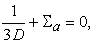(7)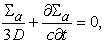(8)

which thus combine to(9)

The differential equation (9) has the following solution (is an integration constant)(10)

From (4) and (7) we see thatand from (10) and (5), respectively, we thus get(11)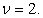(12)

The Lorentz-covariant and dispersion-free quantum transport derived above is thus a Markov process with a multiplication factor of 2, and a cumulative path length distribution function given by the following expression ,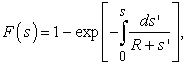(13)

which evaluates to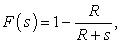(14)

i e a Pareto distribution of the second kind , a distribution more commonly encountered in economics and sociology. The frequency distribution corresponding to the above cumulative path length distribution in (14) is(15)

A typical Pareto frequency distribution of this type is illustrated in Figure 1 and Figure 2.

The path-length distribution above is thus a rational function in contrast to the exponential path-length distribution normally encountered in particle transport theory. In particular - and radically different from normal particle propagation in a medium - the mean free path λ corresponding to the Pareto distribution above will be infinite,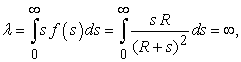(16)

a crucial property thus seeding new clusters very far away.

### 3. Computer Simulations

Figure 3 and Figure 4 show computer simulations in which particles are started at the origin and then followed through successive collisions as given by the Pareto distribution in (14) and (15), and where each collision becomes the starting point for two new trajectories, etc, as described above.

Figure 3. Simulation of particle propagation with Pareto distribution and 12 generations of particle doubling as discussed in the text, showing how the collision probability for the particles close to the source is a short-range, negative exponential, but then further away from the source is a long-range, inverse quadratic function, which leads to new clusters of collision points very far away from the original cluster
Figure 4. Simulation of particle propagation with a Pareto distribution as in Figure 3 but with a different seed for the random number generator, in this case leading to a twin cluster far away from the original cluster and showing a degree of self-similarity with the original system (12 generations of particle doubling)

In these simulations, the path-length distribution is sampled by using a twelve-digit rectangular random number generator for F(s), 0 ≤ F(s) ≤ 1, and with s then(17)

where we have set R = 1.

Figure 5. Quotient Q in (18) plotted as function of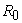andin comparison with QP = 2.55 (light blue plane) as measured  by the Planck space telescope, showing that Q is at least compatible with QP for a range of reasonable values forand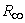. For, e g,= 200 and= 105= 2 107 we thus get Q = 2.67, and for= 300 and=105= 3 107 we get Q = 2.44

As remarked in the Introduction, a quantum doubling of this kind, thus required by Lorentz covariance, very quickly leads to enormous numbers, as is illustrated by the fable  of the inventor of chess. In return for his invention, he is said to have modestly asked for a grain of wheat to be put on the first square of the board, then doubled on the second square, then doubled again on the third square, etc, for each of the 64 squares on the board. His seemingly humble request granted, it then came as a great surprise when it became apparent that so much grain did not by far exist in the entire world. In the simulations performed in this study, the requirements for storage space for the successive generations of new particles similarly limit to around twenty the number of generations that can practically be followed.

As seen from the simulations in Figure 3 and Figure 4, the successive generations of particle creation tend to form very dense clusters of collision points close to each other due to the initial, exponentially falling part of the Pareto distribution. However, in addition to these dense clusters, the inverse quadratic tail of the Pareto distribution, with its infinite mean free path, also seeds far distant parts of the universe with new such clusters. The overall pattern is one with a certain self-similarity , as seen by the enlargements in Figure 3 and Figure 4.

### 4. Ratio of Dark Energy to Dark Matter

From the measurements by the Planck space telescope, the ratio of dark energy to dark matter is calculated as Qp = 68.3% / 26.8% = 2.55 . This ratio should thus be compared to the ratio of the number of quantum trajectories in the simulations described above integrated over all space, relative to the number of quantum trajectories integrated over regions where collision points are accumulated.

However, since the mean free path calculated in (16) above is infinite, the number of dark energy quanta to be considered needs to be confined by some horizon, beyond which no observations can be made. We thus need to determine a typical range, within which the clusters of collision points are mostly confined, and in addition determine a horizon, within which all observable trajectories can be considered to be confined. Using the expression in (16) (with R = 1), we thus want to study the quotient(18)

Assuming that the size of an average galaxy represents the typical rangeof a central cluster of collision points, i e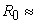105 light years, and that the horizon is at the Hubble radius1010 light years, we then get the ratio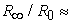105 from astronomical data.

The simulations presented above indicate that a typical rangemight be of the order of 200 units in Figure 3, Figure 4, and Figure 5, which combined with a value= 200 × 105 (with105 from above) in Figure 5 would give a value for the quotient Q in (18) of the order of Q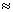2.67, i e of the same order of magnitude as the observed value Qp = 2.55 from the Planck space telescope  as mentioned above.

Above we considered the rangearound an original collision point, and compared the local cluster aroundrelative to all its corresponding far trajectories within the horizon, leading to the quotient Q in (18) above. The same quotient is of course valid also for the total of any set of starting clusters relative to their corresponding far trajectories.

### 5. Concluding Remarks

Instead of trying to speculate on what particles might be involved in the mysterious dark matter and dark energy observed in the universe, the present study starts in the other end and asks what kind of natural requirements on the world around us could possibly result in the creation of something that mass-wise dwarfs everything else in the universe. Once a reasonable candidate for such a mechanism has been identified, then we might perhaps be in a better position to consider the question of whatever particles might be involved.

The present study suggests that Lorentz covariance of the particle propagation, and the resulting, forced, successive particle doubling, might perhaps be the strongest (and possibly only) candidate for the mechanism causing the huge and dominating amounts of dark energy and dark matter that has been observed in the universe.

Although this approach thus leads to a natural and coherent answer why dark energy and dark matter must be there and be so dominating, it still stops short of suggesting what particles might be involved. They would seem to appear like photons, but on the other hand they are also forced by the same Lorentz-covariance to seem (perhaps somewhat unphysically) to double at collisions with the surrounding medium. And all this just in order to obey Lorentz-covariance.

As is discussed in [1, 4], there is actually a corresponding, simpler Galilean world mirroring our world and without this accelerated expansion and particle creation - but that world is not Lorentz-covariant. Not only does Lorentz covariance seem to make our world consistent with regard to observations, but it thus also appears to actually create the very matter that our world is made of (or at least 95 % of it, cf Sect. 1).

So far all attempts have been unsuccessful to identify what particles are involved in the completely dominating dark energy and dark matter content of the universe. It could quite possibly be that the successive quantum multiplications involved in the mechanism studied in this paper lead to a successive degradation of the average energy of the quanta involved to very low levels, and that this fact is part of the reason why they have not yet been detected and identified.

It could of course perhaps also be that trying to answer the question of exactly what particles are involved in dark matter/energy, is a question of the same type as asking exactly what mechanical effect inside clocks moving at relativistic speeds is it that actually causes them to run slow, or exactly what mechanical effect inside atoms is it that actually causes them to become heavier at relativistic velocities. We now know that questions like these are not relevant and impossible to answer, but in the infancy of relativity they were certainly asked.

It could perhaps be that we will have to accept that likewise we may not actually be able to identify the particles seemingly involved in dark energy and dark matter. They produce substantial effects just as the relativistic time-dilation and mass increase do, yes. But what the actual mechanical effect in those historical cases exactly is, and similarly what the actual particles seemingly involved in the present case exactly are, such questions could conceivably be equally irrelevant and impossible to answer.

### Acknowledgements

The author wishes to thank Dr Hans-Olov Zetterström for many fruitful discussions and valuable suggestions over a long period of time with regard to this study. The author is also indebted to the late Dr Staffan Söderberg for valuable mathematical assistance in the initial phase  of this project.

### References

  A. Bergstrom, “Relativistic invariance and the expansion of the universe”, Nuovo Cimento 27B, 145-160 (1975).In article  A. Bergstrom, “Lorentz-covariant quantum transport and the origin of dark energy”, Phys. Scr. 83, 055901 (2011).In article  A. Bergstrom, “Is CMB just an observational effect of a universe in accelerated expansion?”, International Journal of Physics 1, 133-137 (2013).In article  A. Bergstrom, “Dark energy, exponential expansion, CMB, wave-particle duality - all result from Lorentz-covariance of Boltzmann’s transport equation”, International Journal of Physics 2, 112-117 (2014).In article  E. R. Harrison, Cosmology: The Science of the Universe (Cambridge University Press, 2000), Ch 23.In article  http://en.wikipedia.org/wiki/The_Sand_Reckoner retrieved 2014-06-16.In article  http://en.wikipedia.org/wiki/Planck_(spacecraft) retrieved 2014-06-16.In article  A. M. Weinberg and E. P. Wigner, The Physical Theory of Neutron Chain Reactors (Univ. of Chicago Press, 1958), p. 232.In article  J. A. Stratton, Electromagnetic Theory (McGraw-Hill, 1941), p. 550.In article  A. M. Weinberg and E. P. Wigner, The Physical Theory of Neutron Chain Reactors (Univ. of Chicago Press, 1958), p. 235.In article  G. I. Bell and S. Glasstone, Nuclear Reactor Theory (Van Nostrand, 1970), p 54.In article  N. L. Johnson, S. Kotz, and N. Balakrishnan, Continuous Univariate Distributions. vol 1 (Wiley, 1994).In article  http://en.wikipedia.org/wiki/Wheat_and_chessboard_problem retrieved 2014-06-16.In article  A. Bergstrom, “Big Crunch, Big Rip – or a self-similar expansion replenished by dark matter and dark energy?”, International Journal of Physics 2, 146-150 (2014).In article